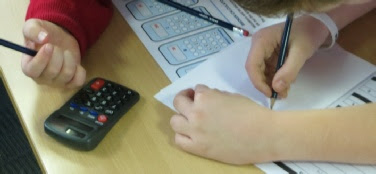School Calendar‎ > ‎Annual Events‎ > ‎

### Maths Challenge 2017

Each year Garsington Primary sends representatives from Year 4 and Year 6 to participate in Maths Challenge events.
This year's events took place at St Edward's School, Oxford. Both Maths Challenge events are a mixture of cerebral exercise and some physical activity but most of all the aim is for the young mathematicians to enjoy taking part.

Year 4 Maths Challenge - Tuesday 7th November 2017

The Year 4 took place at St Edward's School Oxford and included various mathematical tasks including working with dice, pentominoes, playing cards, dominoes, tangrams and counters. The final round posed some multiple choice mathematical problems.

What did our Year 4 mathematicians get up?

Mental Warm Up - Dicey Dicey and Odd One Out

The young mathematicians we asked to find the total of numbers which flashed up on the screen. They were also be given some mathematical facts and had to decide which was the odd one out.

Make 24

There were five examples in this activity where the young mathematicians had to use four given values and four operations to make the answer 24.

Round Robin Activities

These were 8 minute rounds where questions on a certain topic had to be answered. Topics included were:

• Shopping spree
• Puzzles with tangrams or dominoes or pentominoes
• Ordering cards by following given clues
• Mazes including addition mazes where the total has to be reached at the destination
• Simple timetables
• Logic problems

Lateral thinking questions were given with a choice of four answers.  The young mathematicians had to solve the problems and display their winning letters to find out whether they were right or wrong.Year 6 Maths Challenge - 31 October 2017 - 12th out of 39!

The Year 6 event took place at St Edward's School, Oxford and consisted of three challenges.

What challenges did our Year 6 pupils face?

Find the Values

Our young mathematicians were given a set of letters and numbers and by working through ‘equations’ they had to allocate the letters' values. Have a go:

A B C D  =   1 2 3 4

B x C =12

B + D = 6

What are the values of A B C D?

Counting Down!

Given six numbers and knowing they could use an of the usual operators - addition, subtraction, multiplication and division - our Year 6 mathematicians had to work towards making up the total provided.

Like this:

 25 2 10 8 1 7 191

25 x 10 = 250        8 x 7 = 56     2+1 = 3

250-56-3 = 191

The Big Event

A final barrage of mathematical challenges where calculators are not allowed!

How many numbers between 1 and 100 do not have a 9 in them?
How many millimetres in a kilometre?
Ten posts are placed 20 metres apart on a field. What is the distance between the first and last post?
How many different ways are there to give someone 10 pence?
Three numbers add up to 14. Multiplied together they become 84. Find the numbers.
A pen and a pencil cost £5. The pen costs £4 more than the pencil. What is the price of each?
How many spots are there altogether on five normal six-sided dice?
A household has some goldfish, some canaries and rabbits. Altogether there are 15 heads and 26 legs. How many of each animal could there be?